# Area of the shape + Pythagorean theorem - math problems

#### Number of problems found: 229

• Trapezoid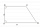The rectangular trapezoid ABCD with right angle at the vertex A has sides a, b, c, d. Calculate the circumference and the area of the trapezoid if given: a = 25cm, c = 10cm, d = 8cm
• Circle inscribed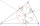There is a triangle ABC and a circle inscribed in this triangle with radius 15. The point T is the point of contact of the inscribed circle with the side BC. What is the area of the triangle ABC if | BT | = 25 a | TC | = 26?
• The triangleThe triangle has sides 5 cm long, 5 cm, 8 cm long. What is the area of the triangle?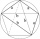The quadrilateral ABCD is composed of two right triangles ABD and BCD. For side lengths: |AD| = 3cm, | BC | = 12cm, | BD | = 5cm. How many square centimeters (area) does the quadrilateral ABCD have? The angles DAB and DBC are right.
• Equilateral triangle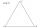Find the area of an equilateral triangle with a side of 15 cm.
• Angle of diagonalsCalculate the perimeter and the area of a rectangle if its diagonal is 14 cm and the diagonals form an angle of 130°.
• The diamondThe diamond has an area S = 120 cm2, the ratio of the length of its diagonals is e: f = 5: 12. Find the lengths of the side and the height of this diamond.
• Side and diagonalFind the circumference and the area of the rectangle if given: side a = 8 cm diagonal u = 10 cm.
• Metal washersMetal washers with a diameter of 80 mm are cut from a strip of steel sheet with a width of 10 cm and a length of 2 m. Calculate the percentage of material waste if no material is lost when two adjacent circles meet.
• Outside pointThe square ABCD and the point E lying outside the given square are given. What is the area of the square when the distance | AE | = 2, | DE | = 5 a | BE | = 4?
• Right isosceles triangle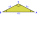What can be the area of a right isosceles triangle with a side length of 8 cm?
• Ratio in trapeziumThe height v and the base a, c in the trapezoid ABCD are in the ratio 1: 6: 3, its content S = 324 square cm. Peak angle B = 35 degrees. Determine the perimeter of the trapezoid
• Ratio of triangles areas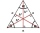In an equilateral triangle ABC, the point T is its centre of gravity, the point R is the image of the point T in axial symmetry, along the line AB, and the point N is the image of the point T in axial symmetry along the line BC. Find the ratio of the area
• Garden GThe rectangular, trapezoidal garden has a base length of 81m, 76m, and a vertical arm of 12m. Calculate how many m2 of the area will remain for planting greenery if 1/3 of the area is built. Calculate the consumption of mesh for land fencing.
• Right triangleA circle with a radius of 5 cm is described in a right triangle with a 6 cm leg. What is the height at the hypotenuse of this triangle?
• Railway embankmentThe railway embankment section is an isosceles trapezoid, the sizes of the bases of which are in the ratio 5: 3. The arms have a length of 5 m, and the height of the embankment is 4.8 m. Calculates the size of the embankment section area.
• Isosceles triangleCalculate the area of an isosceles triangle, the base of which measures 16 cm and the arms 10 cm.
• Isosceles trapezoidFind the area of an isosceles trapezoid, if the bases are 12 cm and 20 cm, the length of the arm is 16 cm
• Diamond area from diagonalsIn the diamond ABCD is AB = 4 dm and the length of the diagonal is 6.4 dm long. What is the area of the diamond?
• The right triangleThe right triangle ABC has a leg a = 36 cm and an area S = 540 cm2. Calculate the length of the leg b and the median t2 to side b.

Do you have an exciting math question or word problem that you can't solve? Ask a question or post a math problem, and we can try to solve it.

We will send a solution to your e-mail address. Solved examples are also published here. Please enter the e-mail correctly and check whether you don't have a full mailbox.

Pythagorean theorem is the base for the right triangle calculator. Examples of area of plane shapes. Pythagorean theorem - math problems.